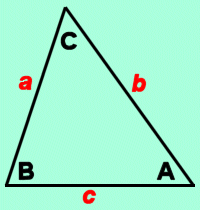Cleave Books
The Triangles Calculator
 Show values to . . . 3 4 5 6 7 8 9 significant figures.
 edge a = units edge b = unitsedge c = units angle A = degrees angle B = degrees angle C = degrees perimeter = units area = square units in-radius = units circum-radius = units
Remember: Appropriate units need to be attached.
Very large and very small numbers appear in e-Format.
Unvalued zeros on all numbers have been suppressed.
The original inputs have NOT been adjusted in any way.
A note on Format and Accuracy is available.

 Additional InformationA triangle is the most basic 2-dimensional shape. It has 3 edges and 3 angles (or vertices).The size of a complete triangle is known as soon as 3 independent pieces of information are given. Independent information means that no one of the 3 pieces given could be found from the other two. For example, giving 3 angles would not be independent information, since any one of them can be worked out from knowing the other 2 (they must all add up to 180 degrees).The position of the information is important. The usual way of ensuring this is as shown in the diagram above. The 3 vertices are identified by 3 capital letters, and the 3 edges opposite those vertices are identified by the same letter as the vertex but written as a lower-case (small) letter.The letters A B C have been used here, but any other 3 letters would do just as well.The In-radius is the radius of the circle which fits just INSIDE the triangle and touches all the edges.The Circum-radius is the radius of the circle which circumscribes the triangle. That is, it fits around the OUTSIDE of the triangle and touches each of the vertices. Ambiguous CaseSometimes TWO triangles can be found to match the given data.This is signalled in the last box with the word AMBIGUOUS. Also it will say "Try supplementary angle at A" (or B or C). Make a note of which angle it is and its size; subtract that size from 180 to make the supplementary angle. Then, after [Clear All] re-enter the same two edge-lengths in their same positions, but enter the supplementary angle in the position suggested (A or B or C).This will then give the details of the alternative triangle which also matches the original data.Note that a triangle can NEVER be ambiguous if     (i) the 3 edges are given (a, b, c)     (ii) two edges and the angle between them are given         (a, b, C) or (a, B c,) or (A, b, c,)     (iii) one edge and any two angles are given.     (iv) one of the given angles is 90 degrees or more. CautionsIf a value of 0 (zero) is given, it means that a solution is possible but is too small to be of any real use.

Go to Cleave Books main Index